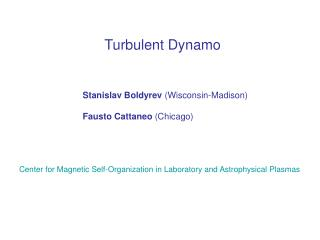DownloadDownload PresentationTurbulent Dynamo

# Turbulent Dynamo

Télécharger la présentation## Turbulent Dynamo

- - - - - - - - - - - - - - - - - - - - - - - - - - - E N D - - - - - - - - - - - - - - - - - - - - - - - - - - -
##### Presentation Transcript

1. Turbulent Dynamo Stanislav Boldyrev (Wisconsin-Madison) Fausto Cattaneo (Chicago) Center for Magnetic Self-Organization in Laboratory and Astrophysical Plasmas

2. Questions 1. Can turbulence amplify a weak magnetic field? • What are the scales and growth rates of • magnetic fields? Kinematic Theory

3. B V1 B V2 MHD Equations tv + (v¢r)v = -rp + (r£B)£B + v + f Kinematic dynamo tB = r£(v£B) + B Re=VL/ - Reynolds number Rm=VL/ - magnetic Reynolds number Pm=/ - magnetic Prandtl number

4. EK K-5/3 K0 K K Kinematic Turbulent Dynamo: Phenomenology V(x, t)is given. tB = r£(v£B) + B Consider turbulent velocity field V(x,t) with the spectrum: V/1/3 » 1/K »/V/-2/3 smaller eddies rotate faster Magnetic field is most efficiently amplified by the smallest eddies in which it is frozen. The size of such eddies is defined by resistivity. B

5. EK K-5/3 EM(K) K0 K K K K Kinematic Turbulent Dynamo: Phenomenology Role of resistivity  Small Prandtl number, Large Prandtl number, PM´/¿ 1 PMÀ 1 Dynamo growth rate: Dynamo growth rate: » 1/ » 1/ “smooth” velocity  V/ “rough” velocity  V/ =1/3

6. EK K-5/3 Large Prandtl number PM=/À 1 Interstellar and intergalactic media EM(K) K0 K K K B  Phenomenology: Large Prandtl Number Dynamo Magnetic lines are folded Cattaneo (1996) Schekochihin et al (2004)

7. EK K-5/3 EM(K) K0 K K K Phenomenology: Small Prandtl Number Dynamo Small Prandtl number PM=/¿ 1 Stars, planets, accretion disks, liquid metal experiments V»1/3 »/V/2/3  / (RM)-3/4 Dynamo growth rate: » 1// (RM)1/2 RM Numerics: Haugen et al (2004) [S.B. & F. Cattaneo (2004)]

8. Kinematic Turbulent Dynamo: Theory V(x, t) is a given turbulent field tB = r£(v£B) + B Two Major Questions: 1. What is the dynamo threshold, i.e., the critical magnetic Reynolds number RM, crit ? 2. What is the spatial structure of the growing magnetic field (characteristic scale, spectrum)? These questions cannot be answered from dimensional estimates!

9. Kinematic Turbulent Dynamo: Theory Dynamo is a net effect of magnetic line stretching and resistive reconnection. RM > RM, crit : stretching wins, dynamo RM < RM, crit : reconnection wins, no dynamo RM=RM, crit: stretching balances reconnection:  B When RMexceeds RM, crit only slightly, it takes many turnover times to amplify the field

10. Kinematic Turbulent Dynamo: Kazantsev Model homogeneity and isotropy incompressibility Dynamo No Dynamo

11. EK K-5/3 EM(K) K0 K K K Kazantsev Model: Large Prandtl Number If we know (r, t), we know growth rate and spectrum of magnetic filed Large Prandtl number: PM=/À 1 • Kazantsev model predicts: • 1. Dynamo is possible; • 2. EM(K)/ K3/2 Numerics agree with both results: Schekochihin et al (2004)

12. EK K-5/3 EM(K) K K0 K K Direct numerical simulations: (2004): No (2007): Yes resolution Kazantsev Model: Small Prandtl Number PM=/¿ 1 Is turbulent dynamo possible? Batchelor (1950): “analogy of magnetic field and vorticity.” No Kraichnan & Nagarajan (1967): “analogy with vorticity does not work.” ? Vainshtein & Kichatinov (1986): Yes

13. EK K-5/3 EM(K) K0 K K K EK K-5/3 EM(K) K K0 K K Small Prandtl Number: Dynamo Is Possible PM=/¿ 1 PM=/À 1 Keep RM constant. Add small-scale eddies (increase Re). Kazantsev model: dynamo action is always possible, but for rough velocity (PM¿1) the critical magnetic Reynolds number (RM=LV/) is very large.

14. Kazantsev Model: Small Prandtl Number L/ Theory S. B. & F. Cattaneo (2004) smooth velocity Kolmogorov (rough) velocity PMÀ 1 PM¿ 1 Simulations P. Mininni et al (2004) A. Iskakov et al (2007) RM, crit May be crucial for laboratory dynamo, PM¿ 1 Re

15. K-5/3 EK Yes, if velocity field has nonzero helicity K0 K K K Kinematic Dynamo with Helicity It is natural to expect that turbulence can amplify magnetic field at K ¸ K0 Can turbulence amplify magnetic field at K ¿ K0 ? “large-scale dynamo”

16. Dynamo with Helicity: Kazantsev Model h=s v¢ (r£ v)d3 x  0 given energy helicity magnetic energy magnetic helicity need to find Equations for M(r, t) and F(r, t) were derived by Vainshtein and Kichatinov (1986)

17. r r Dynamo with Helicity: Kazantsev Model Two equations for magnetic energy and magnetic helicity can be written in the quantum-mechanical “spinor” form: h=s v¢ (r£ v)d3 x = 0 h=s v¢ (r£ v)d3 x  0 S. B. , F. Cattaneo & R. Rosner (2004)

18. Kazantsev model and -model - model assumes scale separation r Kazantsev model, NO scale separation The  - model approaches the exact solution only at r1

19. RM, crit PM 1 Conclusions • Main aspects of kinematic turbulent dynamo is relatively • well understood both phenomenologically and analytically. 2. Dynamo always exists, but 3. Separation of small- and large-scale may be not a correct procedure.